Astroid as a hypocycloid

We have already studied the Ellipsograph or "Trammel of Archimedes". It is a device that consists of a rod that is moving with two fixed points upon two fixed perpendicular lines.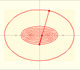An Ellipsograph is a mechanical device used for drawing ellipses.

We know that every point on this rod draws an ellipse.If a straight-line segment is moved in such a way that its extremities travel on two mutually perpendicular straight lines then the midpoint traces out a circle; every other point of the line traces out an ellipse.

If we consider the envelope of this lines of constant length moving with its ends upon two perpendicular lines ("sliding ladder") the result is a curve that has four cups. Its name is Astroid.

The Astroid is also the envelopè of a family of ellipses, the sum of whose axes is constant (the length of the moving segment).The Astroid is the envelope of a segment of constant length moving with its ends upon two perpendicular lines. It is also the envelope of a family of ellipses, the sum of whose axes is constant.

An astroid is defined as the locus of a point P on the circunference of a circle that rolls without slipping inside a larger circle of radius four times as large.

Then the astroid is a kind of hypocycloid. A hypocycloid is a plane curve generated by a fixed point on a small circle that rolls within a large circle.

If the smaller circle has radius r, and the larger circle has radius 4r the curve has four cups. It seems equal to the envelope of the sliding ladder. But, it is the same curve? We need to prove it (following Apostol and Mnatsakanian).

The point P rolls without slipping. The two circular arcs CL and CP have equal lengths because the smaller circle rolls along the larger. The central angle of the smaller circle is four times the central angle of the larger circle (because the radius of the larger circle is four times the radius of the smaller one).How can we draw the tangent to the astroid through P?. We want to probe that segment AB has a fixed length. If we show that the length of AB doesn't change as P moves along the astroid, this will show that AB is a trammel.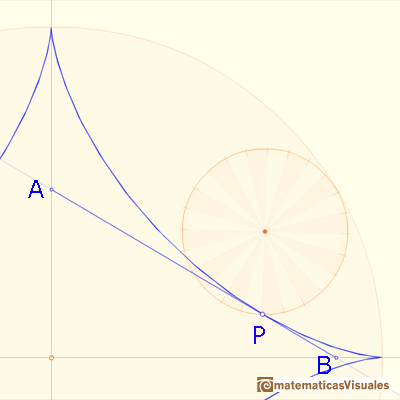M is the midpoint of OC. The line AB drawn through PM, perpendicular to CP, is algo tangent to the astroid at P because C is the center of instantanteous rotation of the smaller circle as it rolls inside the larger circle.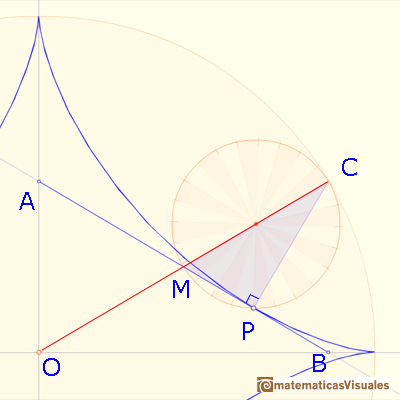To prove that the length of AB doesn't change as P moves along the astroid we can show that M is also the midpoint of AB, and that the triangle OMA is isosceles.

Using the properties of the central and inscribed angles: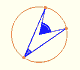Central angle in a circle is twice the angle inscribed in the circle.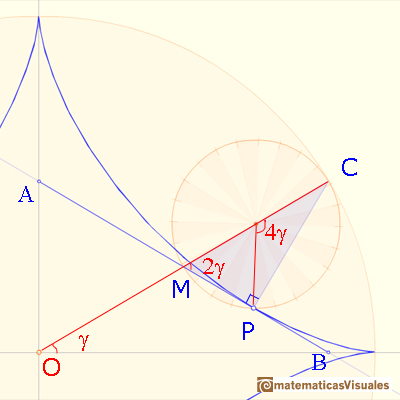An horizontal line through M bisects angle CMA as shown:Then the triangle OMB is isosceles:Triangle OMA is isosceles too. Then AB has fixed length, so it is a trammel, and we have already seen that it is always tangent to the astroid.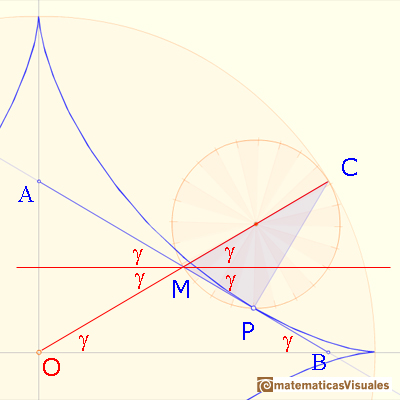"The envelope of a moving trammel of fixed length is an astroid". (Apostol and Mnatsakanian)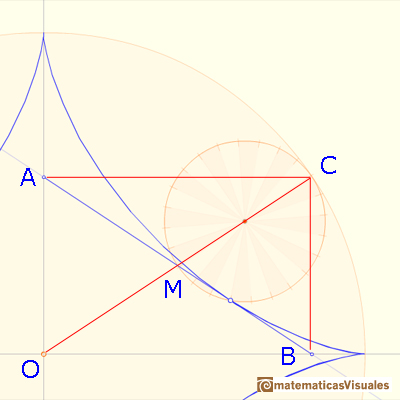REFERENCES

Tom Apostol and Mamikon Mnatsakanian, 'New Horizons in Geometry' (Chapter 9. Trammels, ), Mathematical Association of America, 2012.
H.Martin Cundy and A.P. Rollet, 'Mathematical Models', Oxford University Press, Second Edition, 1961.
Hilbert and Cohn-Vossen, Geometry and the Imagination. Chelsea Publishing Company. pag.278.
Robert C. Yates, 'A Handbook on curves and their properties', J.W.Edwards-Ann Arbor, 1947.
J.L. Coolidge, The Mathematics of great Amateurs. Second Edition. Claredon Press. Oxford. Jan de Witt's proof in page 124.
Historical Mechanisms for Drawing Curves by Daina Taimina. With De Witt's proof why the trammel describes an ellipse.An Ellipsograph is a mechanical device used for drawing ellipses.If a straight-line segment is moved in such a way that its extremities travel on two mutually perpendicular straight lines then the midpoint traces out a circle; every other point of the line traces out an ellipse.Every ellipse has two foci and if we add the distance between a point on the ellipse and these two foci we get a constant.Transforming a circle we can get an ellipse (as Archimedes did to calculate its area). From the equation of a circle we can deduce the equation of an ellipse.In his book 'On Conoids and Spheroids', Archimedes calculated the area of an ellipse. We can see an intuitive approach to Archimedes' ideas.In his book 'On Conoids and Spheroids', Archimedes calculated the area of an ellipse. It si a good example of a rigorous proof using a double reductio ad absurdum.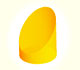We study different cylinders cut by an oblique plane. The section that we get is an ellipse.Plane developments of cones cut by an oblique plane. The section is an ellipse.